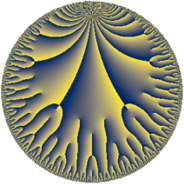# Properties

 Label 16.22.eLevel $16$ Weight $22$ Character orbit 16.e Rep. character $\chi_{16}(5,\cdot)$ Character field $\Q(\zeta_{4})$ Dimension $82$ Newform subspaces $1$ Sturm bound $44$ Trace bound $0$

# Related objects

## Defining parameters

 Level: $$N$$ $$=$$ $$16 = 2^{4}$$ Weight: $$k$$ $$=$$ $$22$$ Character orbit: $$[\chi]$$ $$=$$ 16.e (of order $$4$$ and degree $$2$$) Character conductor: $$\operatorname{cond}(\chi)$$ $$=$$ $$16$$ Character field: $$\Q(i)$$ Newform subspaces: $$1$$ Sturm bound: $$44$$ Trace bound: $$0$$

## Dimensions

The following table gives the dimensions of various subspaces of $$M_{22}(16, [\chi])$$.

Total New Old
Modular forms 86 86 0
Cusp forms 82 82 0
Eisenstein series 4 4 0

## Trace form

 $$82 q - 2 q^{2} - 2 q^{3} + 1604328 q^{4} - 2 q^{5} - 142760912 q^{6} - 5437750796 q^{8} + O(q^{10})$$ $$82 q - 2 q^{2} - 2 q^{3} + 1604328 q^{4} - 2 q^{5} - 142760912 q^{6} - 5437750796 q^{8} - 101897120756 q^{10} + 67333320738 q^{11} + 366511438084 q^{12} - 2 q^{13} + 3790342566044 q^{14} + 4613203124996 q^{15} + 1247007918232 q^{16} - 4 q^{17} + 24726998263422 q^{18} - 46007763621434 q^{19} - 3577794932068 q^{20} + 20920706404 q^{21} - 275054389764220 q^{22} - 568150485016400 q^{24} + 2739706465705224 q^{26} + 1147760716556920 q^{27} - 5464734252855976 q^{28} - 2433173450216602 q^{29} + 1091847142900116 q^{30} - 9835539443769616 q^{31} + 21628424902862408 q^{32} - 4 q^{33} - 32599417569463756 q^{34} - 19253478556034884 q^{35} + 68896817700003052 q^{36} - 7245708527119386 q^{37} - 75508854642654272 q^{38} - 123452072199704568 q^{40} + 521576550189477880 q^{42} + 215332019803969386 q^{43} + 43551785926057540 q^{44} + 953653395699842 q^{45} - 217010424311981172 q^{46} + 1051982644716600976 q^{47} - 791686606171970072 q^{48} - 5585458640832840074 q^{49} + 1308434315006519430 q^{50} + 3238772068175213124 q^{51} - 3929932006670549324 q^{52} + 1889987679210147606 q^{53} + 2474850774763823392 q^{54} + 969847555025010328 q^{56} - 13207633157582037672 q^{58} + 3631979197965016922 q^{59} + 19454740864921105152 q^{60} + 10385362452353654062 q^{61} - 231078435860679632 q^{62} - 17464422381302680956 q^{63} - 28922248017206732928 q^{64} - 9364161130572538716 q^{65} - 1066134537943733516 q^{66} - 17102217044572669130 q^{67} + 1578771419266928720 q^{68} - 3951842785111207532 q^{69} + 37884335466111605120 q^{70} + 50190251198750321124 q^{72} - 146575569503312954228 q^{74} + 145960939174166395366 q^{75} + 205207294437244346196 q^{76} - 82041801652678580764 q^{77} + 18923646523591896860 q^{78} + 204448210818924484480 q^{79} - 129507348099746628056 q^{80} - 802405920297757300870 q^{81} + 830734363602759714528 q^{82} + 317463236552669319678 q^{83} - 1733590905678879817304 q^{84} + 323656241286464843748 q^{85} + 1244418239240036417540 q^{86} + 1366637891097356775928 q^{88} - 4303359727133135334888 q^{90} + 144326756239046079748 q^{91} + 3708288645268614974264 q^{92} - 669717759565183008560 q^{93} - 1146977544252955048048 q^{94} - 1411330138695550130700 q^{95} - 1490348200640250797392 q^{96} - 4 q^{97} + 7549812802452897368090 q^{98} + 2710306841526615010238 q^{99} + O(q^{100})$$

## Decomposition of $$S_{22}^{\mathrm{new}}(16, [\chi])$$ into newform subspaces

Label Dim $A$ Field CM Traces $q$-expansion
$a_{2}$ $a_{3}$ $a_{5}$ $a_{7}$
16.22.e.a $82$ $44.716$ None $$-2$$ $$-2$$ $$-2$$ $$0$$